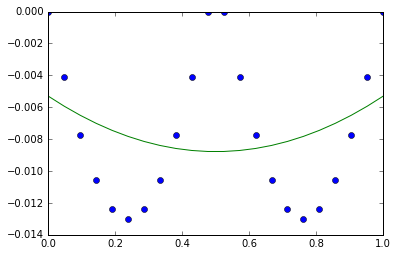# Chapter 13: Statically Indeterminate Problems¶

## Example 13.2 page number 693¶

In :
#Given
#First we will solve without the reaction at middle
#Given
%matplotlib inline
import numpy
import matplotlib.pyplot as plt
import numpy as np
l_ab = 1.0   #2L in - The length of the beam
F_D = 1.0    #W lb/in - The force distribution
F = F_D*l_ab #WL - The force applied
#Beause of symmetry the moment caliculations can be neglected
#F_Y = 0
R_A = F/2 #wl - The reactive force at A
R_B = F/2 #wl - The reactive force at B
#EI - The flxure rigidity is constant and 1/EI =1 # k

#part - A
#section 1--1
l_1 = [0,0.1,0.2,0.3,0.4,0.5,0.6,0.7,0.8,0.9,1] #L taking each section at 0.2L distance
M_1 = [0,0,0,0,0,0,0,0,0,0,0]
v = [0,0,0,0,0,0,0,0,0,0,0]
for i in range(10):
v[i] = R_A - F_D*l_1[i]
M_1[i] = R_A*l_1[i] - F_D*(l_1[i]**2)/2
# (EI)y'' = M_1[i] we will integrate M_1[i] twice where variable is l_1[i]
#(EI)y'-

M_1_intg1 = R_A*(l_1[i]**2)/4 - F_D*(l_1[i]**3)/6 - F_D*(l_ab**3)*l_1[i]/24 #integration of x**n = x**n+1/n+1
#(EI)y- Using end conditions for caliculating constants

M_1_intg2 = R_A*(l_1[i]**3)/12.0 - F_D*(l_1[i]**4)/24.0 + F_D*(l_ab**3)*l_1[i]/24.0
#Equations

l_1 = [0,0.1,0.2,0.3,0.4,0.5,0.6,0.7,0.8,0.9,1] #L taking each section at 0.2L distance
M_1_intg2 = [0,0,0,0,0,0,0,0,0,0,0]
Y = [0,0,0,0,0,0,0,0,0,0,0]
for i in range(10):
M_1_intg2[i] = (l_1[i]**3)/12.0 - (l_1[i]**4)/24.0 - l_1[i]/24.0   # discluding every term for ruling out float values
Y[i] = M_1_intg2[i] #W(l**4)/EI  k = 1/EI
Y_min = 16*min(Y)
print "a) The maximum displacement in y direction is",16*min(Y),"W(l**4)/EI "
print "a) The maximum deflection occured at",2*l_1[Y.index(min(Y))],"L"
f_bb = 2**3/48.0 #l**3/EI - flexibility coefficient
Reac = - Y_min/f_bb #WL , The reaction at the mid of the bar
print "The reaction at the mid of the bar",Reac ,"WL"

#Graphs
Y.extend(Y) #Because of symmetry
values = Y
y = np.array(values)
t = np.linspace(0,1,22)
poly_coeff = np.polyfit(t, y, 2)

plt.plot(t, y, 'o')
plt.plot(t, np.poly1d(poly_coeff)(t), '-')
plt.show()
print "b)The above graph is beam displacement graph"
print "b)The minimum occures in the middle from the above graph  "

a) The maximum displacement in y direction is -0.208333333333 W(l**4)/EI
a) The maximum deflection occured at 1.0 L
The reaction at the mid of the bar 1.25 WLb)The above graph is beam displacement graph
b)The minimum occures in the middle from the above graph


## Example 13.3 page number 694¶

In :
#Given
#First we will solve without the reaction at middle
#Given
import numpy as np
l_ab = 1.0   #2L in - The length of the beam
F_D = 1.0    #W lb/in - The force distribution
F = F_D*l_ab #WL - The force applied
#Beause of symmetry the moment caliculations can be neglected
#F_Y = 0
R_A = F/2 #wl - The reactive force at A
R_B = F/2 #wl - The reactive force at B
#EI - The flxure rigidity is constant and 1/EI =1 # k

#part - A
#section 1--1
l_1 = [0,0.1,0.2,0.3,0.4,0.5,0.6,0.7,0.8,0.9,1] #L taking each section at 0.2L distance
M_1 = [0,0,0,0,0,0,0,0,0,0,0]
v = [0,0,0,0,0,0,0,0,0,0,0]
for i in range(10):
v[i] = R_A - F_D*l_1[i]
M_1[i] = R_A*l_1[i] - F_D*(l_1[i]**2)/2
# (EI)y'' = M_1[i] we will integrate M_1[i] twice where variable is l_1[i]
#(EI)y'-

M_1_intg1 = R_A*(l_1[i]**2)/4 - F_D*(l_1[i]**3)/6 - F_D*(l_ab**3)*l_1[i]/24 #integration of x**n = x**n+1/n+1
#(EI)y- Using end conditions for caliculating constants

M_1_intg2 = R_A*(l_1[i]**3)/12.0 - F_D*(l_1[i]**4)/24.0 + F_D*(l_ab**3)*l_1[i]/24.0
#Equations

l_1 = [0,0.1,0.2,0.3,0.4,0.5,0.6,0.7,0.8,0.9,1] #L taking each section at 0.2L distance
M_1_intg2 = [0,0,0,0,0,0,0,0,0,0,0]
Y = [0,0,0,0,0,0,0,0,0,0,0]
for i in range(10):
M_1_intg2[i] = (l_1[i]**3)/12.0 - (l_1[i]**4)/24.0 - l_1[i]/24.0   # discluding every term for ruling out float values
Y[i] = M_1_intg2[i] #W(l**4)/EI  k = 1/EI
e_1 = 16*min(Y)         #WL4/EI - The maximum defection
e_2 = - F_D*((2*l_ab)**3)/24.0 #WL3/EI - The maximum angle
#Caliculating for momentum and force
f_ab = ((2*l_ab)**2)/16.0 #L2/EI
f_bb = ((2*l_ab)**3)/48.0 #L3/EI
f_aa = 2*l_ab/3.0 #L/EI
f_ba = ((l_ab)**2)/4.0 #L2/EI
#F*X = e - Matrix multiplication
#Solving for X
a = np.array([[f_aa,f_ba], [f_ba,f_bb]])
b = np.array([e_2,e_1])
x = np.linalg.solve(a, b)
print "The reactive moment at A i.e M_A",x,"WL**2"
print "The reactive force at A i.e R_A",x,"WL"

The reactive moment at A i.e M_A -0.0714285714286 WL**2
The reactive force at A i.e R_A -1.14285714286 WL

In [ ]: